# Ornstein-Zernike relation from the grand canonical distribution function

Defining the local activity by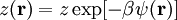$z({\mathbf r})=z\exp[-\beta\psi({\mathbf r})]$

where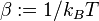$\beta:=1/k_BT$, and$k_B$ is the Boltzmann constant. Using those definitions the grand canonical partition function can be written as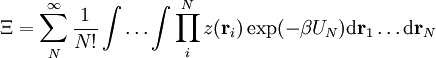$\Xi=\sum_N^\infty{1\over N!}\int\dots\int \prod_i^Nz({\mathbf r}_i)\exp(-\beta U_N){\rm d}{\mathbf r}_1\dots{\rm d}{\mathbf r}_N$.

By functionally-differentiating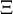$\Xi$ with respect to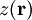$z({\mathbf r})$, and utilizing the mathematical theorem concerning the functional derivative,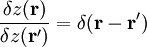${\delta z({\mathbf r})\over{\delta z({\mathbf r'})}}=\delta({\mathbf r}-{\mathbf r'})$,

we obtain the following equations with respect to the density pair correlation functions: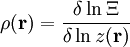$\rho({\mathbf r})={\delta\ln\Xi\over{\delta \ln z({\mathbf r})}}$,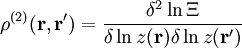$\rho^{(2)}({\mathbf r},{\mathbf r}')={\delta^2\ln\Xi\over{\delta \ln z({\mathbf r})\delta\ln z({\mathbf r'})}}$.

A relation between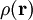$\rho({\mathbf r})$ and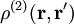$\rho^{(2)}({\mathbf r},{\mathbf r}')$ can be obtained after some manipulation as,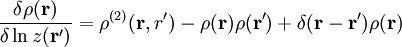${\delta\rho({\mathbf r})\over{\delta \ln z({\mathbf r'})}}=\rho^{(2)}({\mathbf r,r'})-\rho({\mathbf r})\rho({\mathbf r'})+\delta({\mathbf r}-{\mathbf r'})\rho({\mathbf r})$.

Now, we define the direct correlation function by an inverse relation of the previous equation,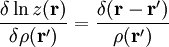${\delta \ln z({\mathbf r})\over{\delta\rho({\mathbf r'})}}={\delta({\mathbf r}-{\mathbf r'})\over{\rho({\mathbf r'})}}$.

Inserting these two results into the chain-rule theorem of functional derivatives,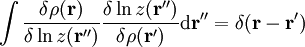$\int{\delta\rho({\mathbf r})\over{\delta \ln z({\mathbf r}^{\prime\prime})}}{\delta \ln z({\mathbf r}^{\prime\prime})\over{\delta\rho({\mathbf r'})}}{\rm d}{\mathbf r}^{\prime\prime}=\delta({\mathbf r}-{\mathbf r'})$,

one obtains the Ornstein-Zernike relation. Thus the Ornstein-Zernike relation is, in a sense, a differential form of the partition function. (Note: the material in this page was adapted from a text whose authorship and copyright status are both unknown).